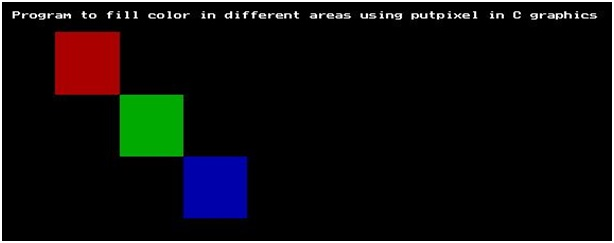# Design a concentric circle of graphics.h in C

In this article, we are going to learn about the use of co-ordinates and radius to create a circle by passing these two things as a parameter in a pre-defined circle() function and the process to use for loop for creating the concentric circles.
Submitted by Manu Jemini, on March 24, 2018

Why is it important to make circles and circles? It’s because we want to create a pattern which is not there to draw by the built-in functions of our graphics.h library.

One way to look at it is that we are using a simple function to create a complex shape. This shape can be changed easily because we are responsible for creating it in such a way that it looks like a steel cylinder with a hole.

How to create it? All we need to is to make a loop and iterate in a range and while iterating through the range pass down the range as the radius. The Trick is that we will create circles of increasing or decreasing radius on the same center.

This will do the job.

## graphics.h - Design a concentric circle in C

﻿

```#include <graphics.h>
#include <conio.h>

int main()
{
//initilizing graphic driver and
//graphic mode variable
int graphicdriver=DETECT,graphicmode;

//calling initgraph
initgraph(&graphicdriver,&graphicmode,"c:\\turboc3\\bgi");

//Printing message for user
outtextxy(20, 20 + 20, "Program to create a concentric circle in C graphics");

//initilizing the variables
int x = 200, y = 200, a;

//for loop to create a circle
for ( a = 25; a <= 100 ; a = a + 3)
circle(x, y, a);

getch();

return 0;
}
```

Output